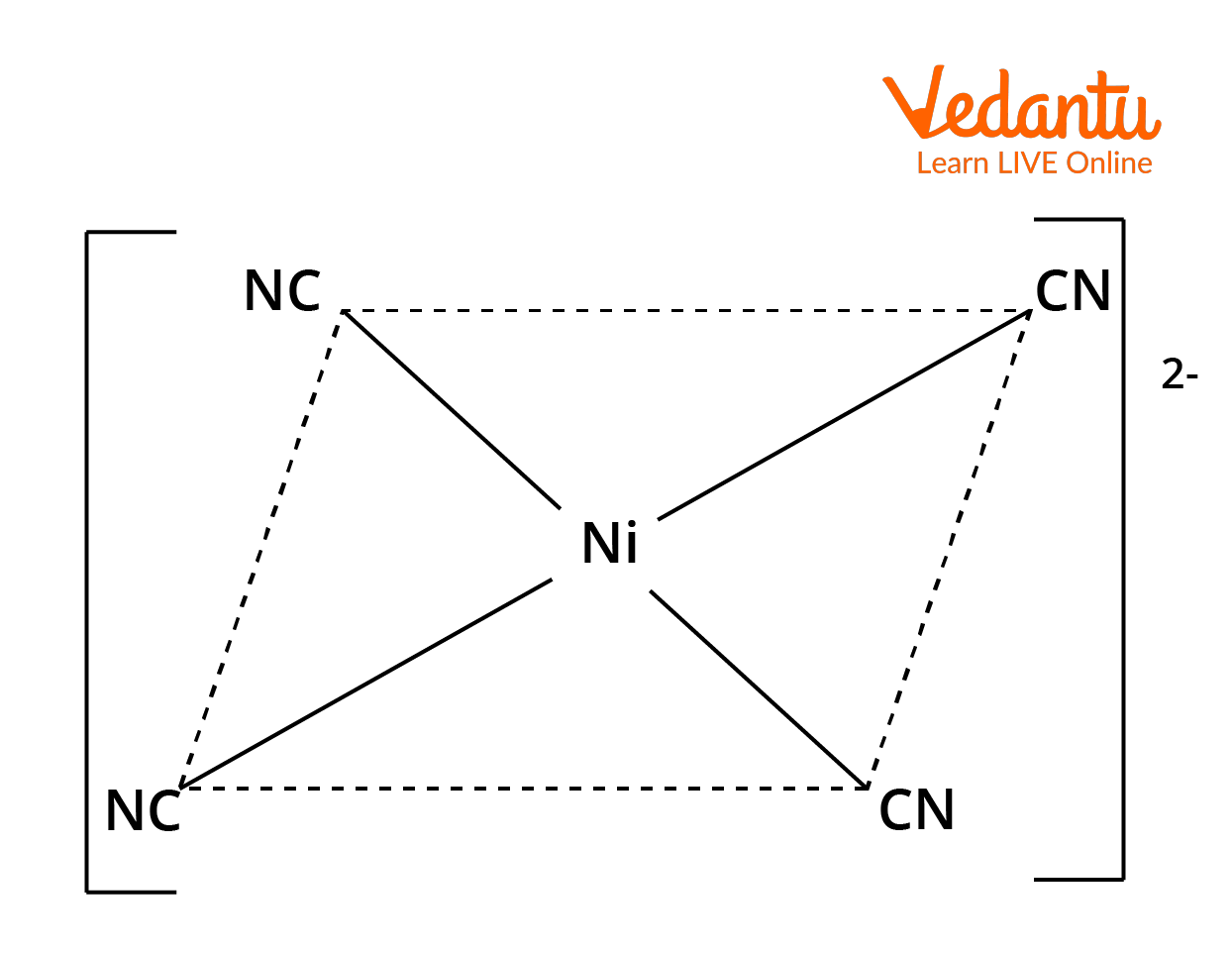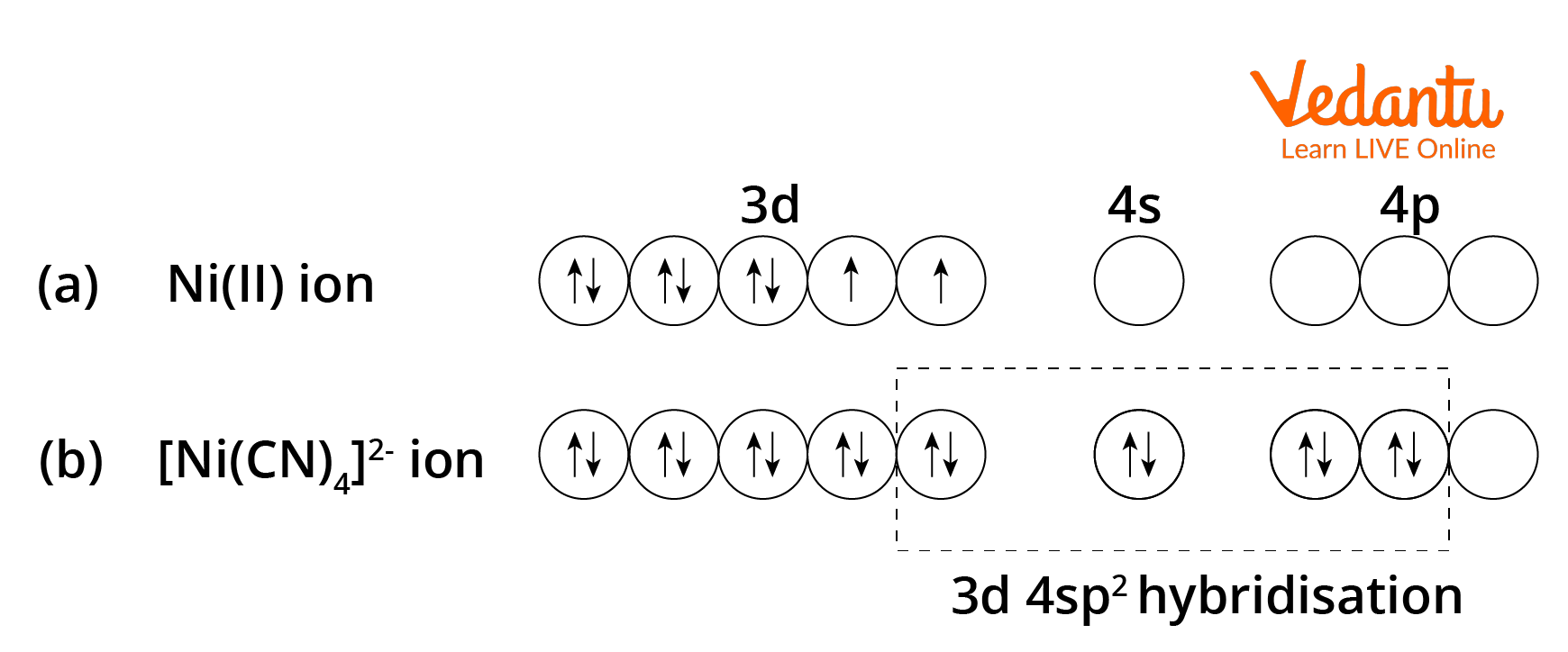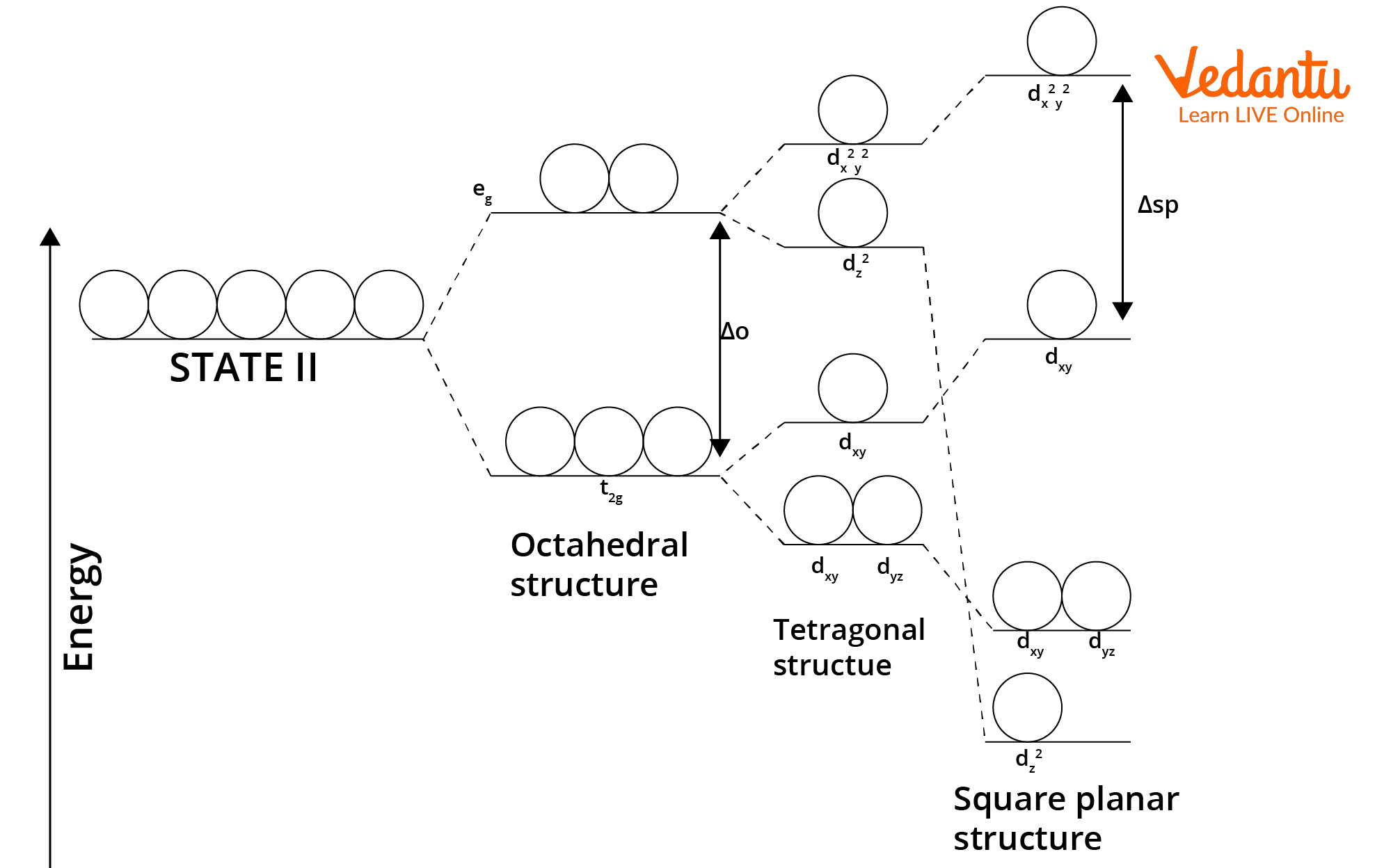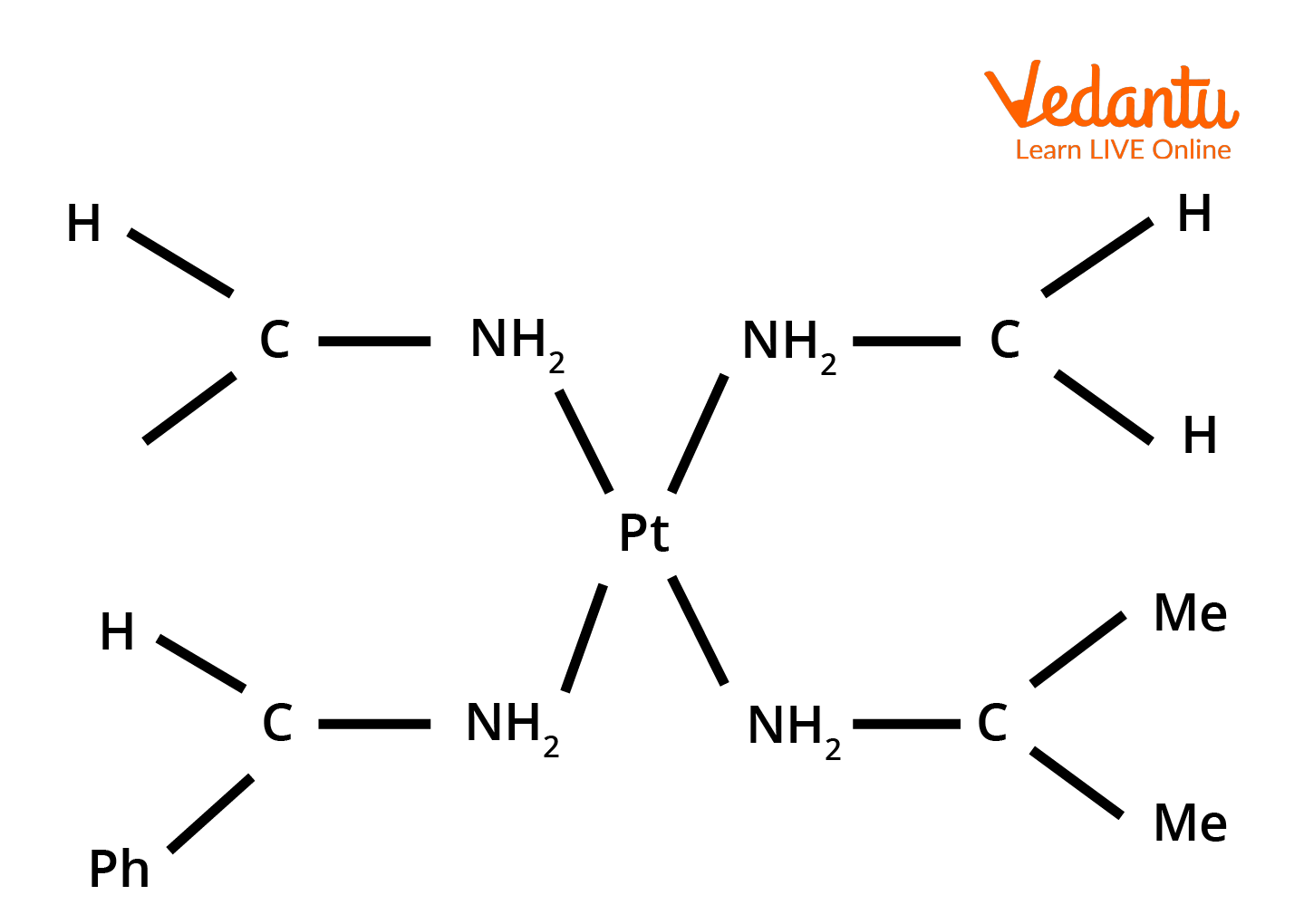Courses
Courses for Kids
Free study material
Free LIVE classes
More

# Square Planar Complex for JEELIVE
Join Vedantu’s FREE Mastercalss

## Introduction to Coordination Complexes

A coordination complex may be defined as a compound that results from the combination of two or more stable chemical species and retains its identity in the solid as well as in the dissolved state. A coordination compound that is soluble in water almost invariably contains a complex ion. Thus, [Cu(NH3)4]SO4 contains the complex ion [Cu(NH3)4]2+.

## Square Planar Geometry of Coordination Compounds

Now we have to answer which complex has square planar structure. Square planar geometry is common amongst the complexes of transition elements only. In square planar molecular geometry, the central atom will be surrounded by the constituent atoms, which form the corners of a square on the same plane. The geometry is common for transition metal complexes with a d8 configuration.

The common examples of this type of geometry are [PtCl4]2-, [NiCN4]2-, [PdCl4]2-, etc. The geometry of [NiCN4]2- is shown in the Image: 1.Square Planar Geometry of  [NiCN4]2-

## Formation of Square Planar Complexes

In this category, we shall consider the nickel complexes in which the coordination number of the metal ion is 4. The typical example is offered by [Ni(CN)4]2-.

## Tetracyanonickelate (II) ion, [Ni(CN)4]2-.

The Nickel (II) ion has 3d8 as its outer electronic configuration, and according to Hund’s rule of maximum multiplicity, it will have two unpaired electrons, as shown in image 2. Depending upon the type of hybridization involved, there are two probable ways in which the complexes of Nickel with coordination number 4 that may be formed. If the complexe involves 4sp3 hybridization, it would have a tetrahedral structure. If, on the other hand, the complex involves 3d4sp2 hybridization, it would have a square planar structure.

For the formation of a square planar structure through dsp2 hybridization, one of the 3d orbitals should be empty and available for hybridisation. This is possible if the unpaired d electrons are paired up with the help of energy made available by the approach of ligands, thereby making one of the 3d orbitals empty. There would thus be 0 unpaired electrons and the complex would be diamagnetic.

We know from experiment that the complex ion [Ni(CN)4)2- is diamagnetic, indicating that the hybridisation involved in this case is 3d4sp2 as represented in image 2. Consequently, the structure and shape of the complex would be of square planar hybridization. The empty hybrid orbitals of cyanide ions to form metal ligand coordinate bonds, as shown in the image. The dotted arrows represent the electron pairs donated by the CN- ions.Formation of Square Planar Hybridization [Ni(CN)4]2- Complex, Involving 3d4sp2 Hybridization of Ni2+ ion orbitals.

## Crystal Field Splitting in Square Planar Complexes

The splitting of d orbitals in the square planar complexes can be understood by gradually withdrawing two ligands lying along the Z axis from the octahedral complex.

As the ligands lying on the Z axis plane are moved away, the ligands in the XY plane tend to approach the central ion more closely. As a result, the electrons of d orbitals in the XY Plane experience greater repulsion from the electrons of ligands in a square planar complex than in an octahedral complex. This causes an increase in the energy of d orbitals in the XY Plane, i.e., an increase in the energy of \$d_{x^2 - y^2}\$ and dxy orbitals in square planar complexes compared to their energies in octahedral complexes as illustrated in image 3.

Furthermore, since the ligands lying on the Z axis have been moved away from the plane, the electrons in the d orbitals along the Z axis as well as in the XZ and YZ planes experience relatively smaller replusions from the electrons of the ligands. This results in an appreciable fall in the energy of \$d_{z^2}\$ orbitals as well as dxz and dyz orbitals.

As the trans ligands lying along the Z axis drop out completely, a square planar complex ML4 is formed. This is accompanied by a further rise in the energies of \$d_{x^2 - y^2}\$ and dxy orbitals and a further fall in the energies of \$d_{z^2}\$, dxz and dyz orbitals, as illustrated in the figure. The crystal field splitting in the case of a square planar complex is indicated by \$\Delta_{sp}\$. The d orbital used in square planar complex are as follows:Crystal Field Splitting in the Square Planar Complexes.

## Conclusion

The electronic distribution in the metal will not be symmetrical because of an orbital which is only half filled (either the \$d_{x^2 - y^2}\$  or \$d_{z^2}\$). If it is \$d_{x^2 - y^2}\$  orbital, then the nucleus of the metal is less affected by the ligand field along the x and y directions than along the z direction. Again, if the ligands are considered to be situated at the corners of a regular octahedron, those in the XY plane will be more attracted than those along the z axis. We also came across that which complex has square planar structure and also about the d orbital used in square planar complex.

This asymmetrical distribution of electrons thus results in four equivalent bonds (shorter) of one kind and two equivalent bonds (longer) of another kind in an octahedral field. If it were the \$d_{x^2}d_{z^2}\$ orbitals, which contain one unpaired electron, the exact opposite would happen, and we would get two shorter and four larger bonds in the octahedral complex.

The square planar complexes are thus a special case of the octahedral complexes when two bonds are extended too much, resulting in an unsymmetrical electronic distribution on the metal.

Last updated date: 26th Sep 2023
Total views: 2.7k
Views today: 0.02k

## FAQs on Square Planar Complex for JEE

1.  Why don’t square planar complexes show optical isomerism?

Square Planar complexes are not optically active. This is because all the ligands and the central metal atom lie in the same plane. The molecule has a plane of symmetry (the plane of the molecule is the plane of the symmetry) and this cannot exhibit optical isomerism. One such compound, which has, however, been resolved into two forms, is shown in the image. Generally, square planar complexes do not show optical isomerism since they have no plane of symmetry.Asymmetrical Molecule of Square Planar Geometry

2. Which complex has a molecule of Square Planar shape  out of the following?

1. [MnCl4]2- (b) [CuCl4]2- (c) [FeF6]3- (d) [Cu(NH3)4]2+

(d)  [Cu(NH3)4]2+

The complex [MnCl4]2- has 4sp3 hybridization and a tetrahedral geometry.  [CuCl4]2- also has 4sp3 hybridization and a tetrahedral geometry.  [FeF6]3- has 4sp3d2 hybridization and octahedral geometry. The complex  [Cu(NH3)4]2+ has the electronic configuration of 3d4sp2 hybridization and coordination bonds are formed. It has 1 unpaired electron and an oxidation number of +2. Also due to the presence of an unpaired electron, the complex [Cu(NH3)4]2+ will be paramagnetic. Thus, it forms a square planar shape.